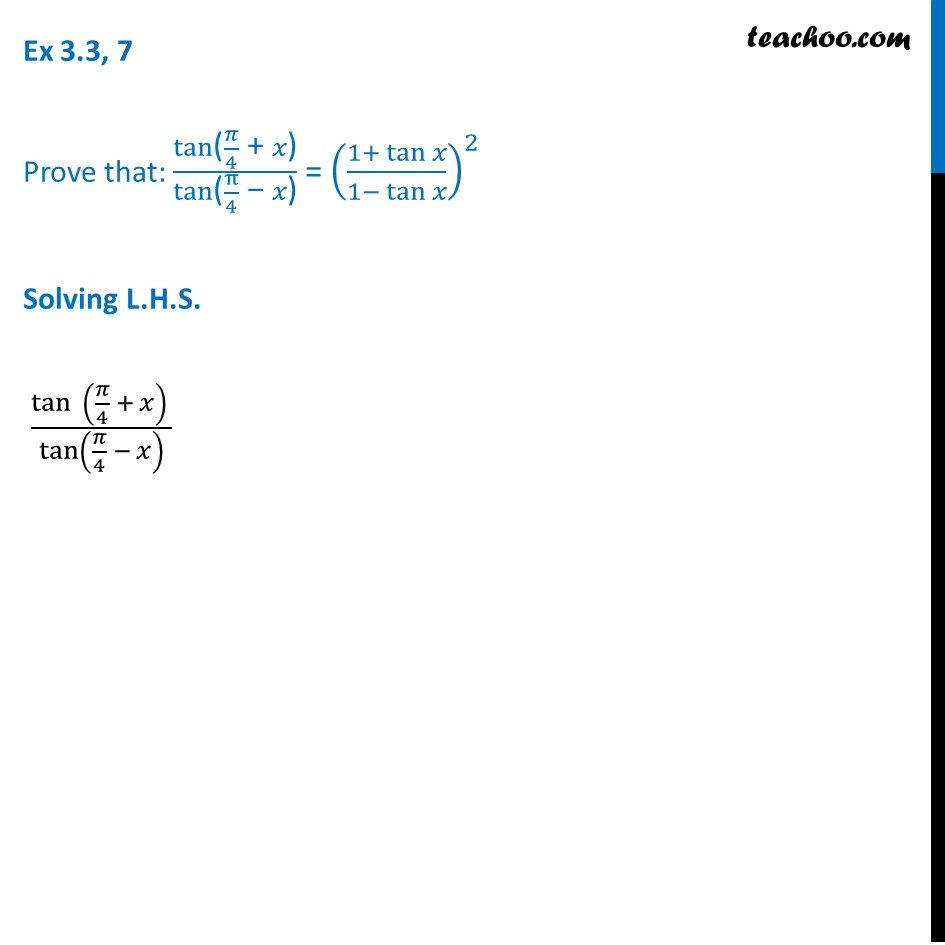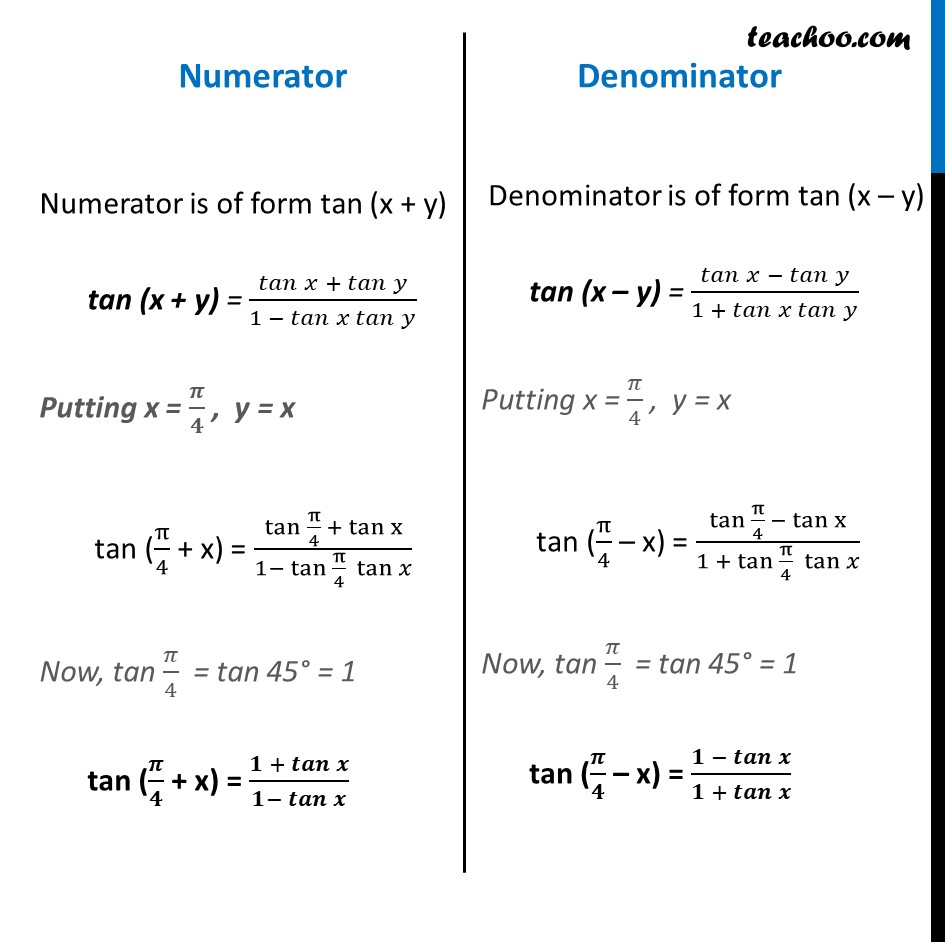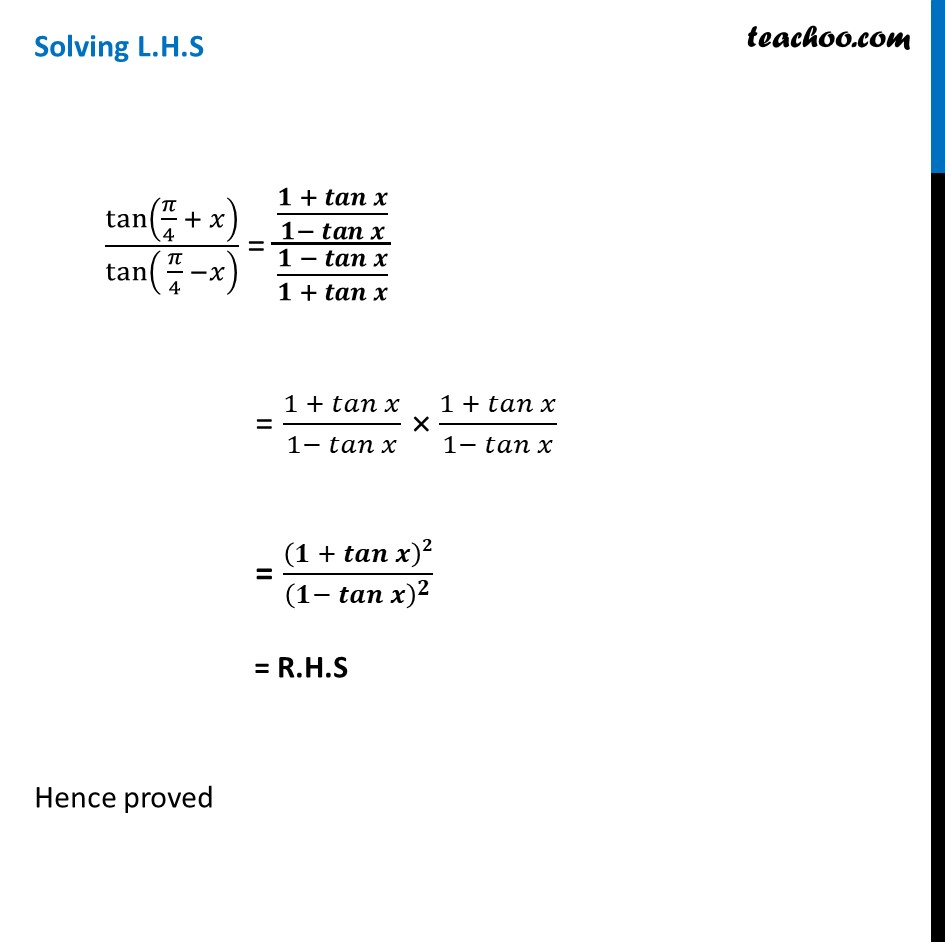Ex 3.3

Chapter 3 Class 11 Trigonometric Functions
Serial order wiseLearn in your speed, with individual attention - Teachoo Maths 1-on-1 Class

### Transcript

Ex 3.3, 7 Prove that: (tan"(" 𝜋/4 " + " 𝑥")" )/(tan"(" π/4 " − " 𝑥")" ) = ((1+ tan" " 𝑥)/(1− tan" " 𝑥))^2 Solving L.H.S. (tan⁡ (𝜋/4 + 𝑥) )/tan⁡(𝜋/4 − 𝑥) Numerator Numerator is of form tan (x + y) tan (x + y) = (𝑡𝑎𝑛" " 𝑥 + 𝑡𝑎𝑛⁡𝑦)/(1 − 𝑡𝑎𝑛 𝑥 𝑡𝑎𝑛⁡𝑦 ) Putting x = 𝝅/𝟒 , y = x tan (π/4 + x) = (tan π/4 + tan⁡x)/(1− tan π/4 tan⁡𝑥 ) Now, tan 𝜋/4 = tan 45° = 1 tan (𝝅/𝟒 + x) = (𝟏 + 𝒕𝒂𝒏⁡𝒙)/(𝟏− 𝒕𝒂𝒏⁡𝒙 ) Denominator Denominator is of form tan (x – y) tan (x – y) = (𝑡𝑎𝑛" " 𝑥 − 𝑡𝑎𝑛⁡𝑦)/(1 + 𝑡𝑎𝑛 𝑥 𝑡𝑎𝑛⁡𝑦 ) Putting x = 𝜋/4 , y = x tan (π/4 – x) = (tan π/4 − tan⁡x)/(1 + tan π/4 tan⁡𝑥 ) Now, tan 𝜋/4 = tan 45° = 1 tan (𝝅/𝟒 – x) = (𝟏 − 𝒕𝒂𝒏⁡𝒙)/(𝟏 + 𝒕𝒂𝒏⁡𝒙 ) Solving L.H.S tan⁡(𝜋/4 + 𝑥)/tan⁡( 𝜋/4 −𝑥) = ((𝟏 + 𝒕𝒂𝒏⁡𝒙)/(𝟏− 𝒕𝒂𝒏⁡𝒙 ))/((𝟏 − 𝒕𝒂𝒏⁡𝒙)/(𝟏 + 𝒕𝒂𝒏⁡𝒙 )) = (1 + 𝑡𝑎𝑛⁡𝑥)/(1− 𝑡𝑎𝑛⁡𝑥 ) × (1 + 𝑡𝑎𝑛⁡𝑥)/(1− 𝑡𝑎𝑛⁡𝑥 ) = (𝟏 + 𝒕𝒂𝒏⁡𝒙 )𝟐/(𝟏− 𝒕𝒂𝒏⁡𝒙 )^𝟐 = R.H.S Hence proved